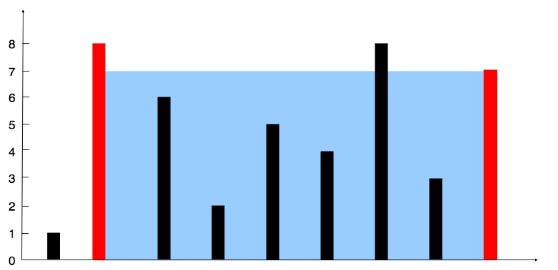# # 11. 盛最多水的容器

11. 盛最多水的容器open in new window``````输入：[1,8,6,2,5,4,8,3,7]

``````

``````class Solution {
public int maxArea(int[] height) {
int maxArea = 0;
int left = 0;
int right = height.length - 1;

while(left < right) {
maxArea = Math.max(maxArea, Math.min(height[left], height[right]) * (right - left));
if(height[left] > height[right]) {
right--;
} else {
left++;
}
}

return maxArea;
}
}
``````